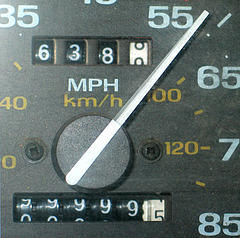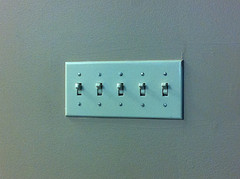# Assignment 3 – circuit diagrams

due at 23:59 on   +50

# Truth table

Write a complete truth table for the values of the Boolean expression

$$A\cdot C + B\cdot C + C'\cdot(A+B)$$

Remember the correct order of operations: parentheses, negation (NOT), then AND, then OR.

You may create your table on paper and then take a very clear picture of it, or do it directly in a document or spreadsheet file.

# Circuit 1

Implement a circuit for the above Boolean expression, using Logisim. It should have three two-state pins, labeled as “A”, “B”, and “C”. It should have one LED. Test the circuit against your truth table to verify the results. If you find a way to implement the same expression with fewer gates, that’s fine (but not necessary). Save your work as truth1.circ and upload that file to the dropbox (link below) to submit.

# Circuit 2: Gray converter

One feature of positional numbering systems is what I like to call the odometer effect. This is what happens when a mechanical mileage counter on a car reads something like 9999 and then “flips over” to 10000. All the dials have to turn at the same time.A mechanical car odometer [mulears on Flickr]

The odometer effect happens much more often in binary. Incrementing any odd number ends up flipping more than one bit at a time. Here are some examples:

 increment 3 to 4:  011 to  100 (3 bits change)
increment 5 to 6:  101 to  110 (2 bits change)
increment 7 to 8: 0111 to 1000 (4 bits change)

In some electro-mechanical systems, flipping so many bits on every increment can be awkward – perhaps it uses a lot of power, or it produces excessive vibration. Imagine counting through all 32 configurations of a sequence of 5 light switches. These transitions would slow you down!An array of switches [randomcuriosity on Flickr]

In 1953, Frank Gray of Bell Labs developed a “reflected binary code” (now called Gray code), to avoid the odometer effect when working with binary sequences.

A two-bit Gray code sequence looks like this:

  00
01
11
10

That sequence covers all the two-bit binary numbers, although in a strange order. But there is exactly one bit that changes when transitioning from one number to the next, and back around to the beginning.

00 (flip the right bit) → 01 (then flip left bit) → 11 (then flip right again) → 10 (flip left again) → 00 (back at the beginning).

To devise sequences with more bits, you can mirror-image a shorter sequence (which is why it’s called a “reflected” binary code). That is, start with the two-bit sequence above, then write it backwards:

  00  (original two-bit sequence)
01
11
10
10  (same sequence in reverse order)
11
01
00

Then fill in zeroes to the left of the first half, and ones next to the right half.

  000  (first half starts with zeroes)
001
011
010
110  (second half starts with ones)
111
101
100

Now you have the 3-bit Gray code. Again, only one bit changes when going from any number to the next, so there is no odometer effect.

Your task is to build a circuit that allows me to click pins to enter 4-bit gray codes, and convert that to the corresponding binary number. Check out how it will work in this 30-second video.

gray-hex

I am clicking one gray-code bit at a time. The LEDs at the bottom are lighting up in standard binary order. To emphasize that, I also used a seven-segment hexadecimal display to cycle through the numbers 0-9 then A-F.

You can start with my template file: gray-hex-start.circ which already contains the pins, LEDs, and hex display. It just omits the gates from the part shown as “censored” in the video.

Here are the formulas governing Gray-to-binary translation:

• B3 = G3
• B2 = B3 ⊕ G2
• B1 = B2 ⊕ G1
• B0 = B1 ⊕ G0

You can find the complete 4-bit Gray sequence on the Gray code wikipedia page.

Save your work as gray2.circ. Upload all your files for this assignment to the dropbox at https://www.dropbox.com/request/Z9QeGmGb3NH8VfCs7vPm.